# Binary Counters

## Binary Counters

Normally binary counters are used for counting the number of pulses coming at the input line in a specified time period.

The binary counters must possess memory since it has to remember its past states.

As the name suggests, it is a circuit which counts.The main purpose of the counter is to record the number of occurrence of some input.

Counters can be classified into two broad categories according to the way they are clocked:
1. Asynchronous (Ripple) Counters – The first flip-flop is clocked by the external clock pulse, and then each successive flip-flop is clocked by the Q or Q’ output of the previous flip-flop.

2. Synchronous Counters – All memory elements are simultaneously triggered by the same clock.

### Asynchronous or Ripple Counters

A two-bit asynchronous counter is shown below in fig.1. The toggle(T) flip-flops are being used. But we can use the JK flip-flop also with J and K connected permanently to logic 1.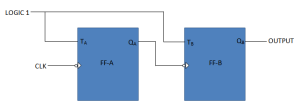Fig.1

The external clock is connected to the clock input of the first flip-flop (FF-A) only and  QA output is applied to the clock input of the next flip-flop i.e. FF-B.

So, FF-A changes state at the falling edge of each clock pulse, but FF-B changes only when triggered by the falling edge of the QA output of FF-A.

Because of the inherent propagation delay through a flip-flop, the transition of the input clock pulse and a transition of the QA output of FF-A can never occur at exactly the same time.

Therefore, the flip-flops cannot be triggered simultaneously, producing an asynchronous operation.

### Operation

Initially let both the Flip-flops be in the reset state i.e QAQA= 00

After 1st negative clock edge:

• As soon as the first negative clock edge is applied to FF-A, QA will be equal to 1.
• QA is connected to clock input of FF-B. Since QA has changed from 0 to 1, it is treated as the positive clock edge by FF-B.  So, there is no change in QB because FF-B is a negative edge triggered FF.

Hence, QBQA = 01…………….After the first clock pulse

After 2nd negative clock edge:

• On the arrival of second negative clock edge, FF-A toggles again and QA = 0.
• The change in QA acts as a negative clock edge for FF-B. So it will also toggle, and QB will be 1.

Hence, QBQA = 10…………….After the second clock pulse

After 3rd negative clock edge:

• On the arrival of 3rd negative clock edge, FF-A toggles again and QA become 1 from 0.
• Since this is a positive going change,FF-B does not respond to it and remains inactive. So QB does not change and continues to be equal to 1.

Hence, QBQA = 11…………….After the third clock pulse

After 4th negative clock edge:

• On the arrival of 4th negative clock edge, FF-A toggles again and QA become 1 from 0.
• This negative change in QA acts as clock pulse for FF-B. Hence it toggles to change QB from 1 to 0.

Hence, QBQA = 00…………….After the fourth clock pulse

### Synchronous counters

If the “clock” pulses are applied to all the flip-flops in a counter simultaneously, then such a counter is called as synchronous counter.

### 2-Bit Synchronous UP Counter

The JA and KA inputs of FF-A are tied to logic 1. So FF-A will work as a toggle flip-flop. The JB and Kinputs are connected to QA.

#### Logic Diagram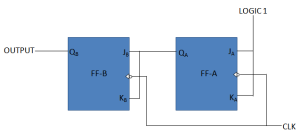Fig.2

#### Operation

Initially let both the FFs be in the reset state:

QBQA = 00…………….initially

After 1st negative clock edge

• As soon as the first negative clock edge is applied, FF-A will toggle and QA will change from 0 to 1.
• But at the instant of application of negative clock edge, QA ,JB = KB =0 Hence FF-B will not change its state. So QB will remain 0.

Hence, QBQA = 01…………….After the first clock pulse

After 2nd negative clock edge

• On the arrival of second negative clock edge, FF-A toggles again and QA change from 1 to 0.
• But at this instant QA was 1. So JB = KB=1 and FF-B will toggle. Hence QB changes from 0 to 1.

Hence, QBQA = 10…………….After the second clock pulse

After 3rd negative clock edge

• On application of the third falling clock edge, FF-A will toggle from 0 to 1 but there is no change of state for FF-B.

Hence, QBQA = 11…………….After the third clock pulse

After 4th negative clock edge

• On application of the next clock pulse, QA will change from 1 to 0 as QB will also change from 1 to 0.

Hence, QBQA = 00…………….After the fourth clock pulse

### Classification of counters

Depending on the way in which the counting progresses, the synchronous or asynchronous counters are classified as follows.

• Up counters
• Down counters
• Up/Down counters

### UP/DOWN Counter

In the up/down counter, up counter and down counter are combined together to obtain an UP/DOWN counter.

A mode control (M) input is also provided to select either up or down mode.

A combinational circuit is required to be designed and used between each pair of flip-flop in order to achieve the up/down operation.

Type of up/down counters:

• UP/DOWN ripple counters
• UP/DOWN synchronous counters

### UP/DOWN Ripple Counters

In the UP/DOWN ripple counter all the FFs operate in the toggle mode.

So either T flip-flops or JK flip-flops are to be used.

The LSB flip-flop receives clock directly. But the clock to every other FF is obtained from (Q or Q bar) output of the previous FF.

• UP counting mode (M=0):  The Q output of the preceding FF is connected to the clock of the next stage if up counting is to be achieved. For this mode, the mode select input M is at logic 0 (M=0).
• DOWN counting mode (M=1) :  If M =1, then the Q bar output of the preceding FF is connected to the next FF. This will operate the counter in the down counting mode.

#### EXAMPLE

3-bit binary up/down ripple counter.

• 3-bit : hence three FFs are required.
• UP/DOWN : So a mode control input is essential.
• For a ripple up counter, the Q output of preceding FF is connected to the clock input of the next one.
• For a ripple down counter, the Q bar output of preceding FF is connected to the clock input of the next one.
• Let the selection of Q and Q bar output of the preceding FF be controlled by the mode control input M such that, If M = 0, UP counting. So connect Q to CLK. If M = 1, DOWN counting. So connect Q bar to CLK.

#### BLOCK DIAGRAMFig.3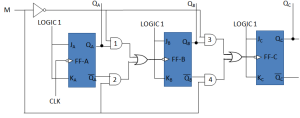Fig.4

#### TRUTH TABLE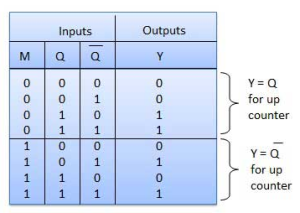#### OPERATION

Case 1: With M = 0 (Up counting mode)

• If M = 0 and M bar = 1, then the AND gates 1 and 3 in fig. will be enabled whereas the AND gates 2 and 4 will be disabled.
• Hence QA gets connected to the clock input of FF-B and QB gets connected to the clock input of FF-C.
• These connections are same as those for the normal up counter. Thus with M = 0 the circuit work as an up counter.

Case 2: With M = 1 (Down counting mode)

• If M = 1, then AND gates 2 and 4 in fig. are enabled whereas the AND gates 1 and 3 are disabled.
• Hence QA bar gets connected to the clock input of FF-B and QB bar gets connected to the clock input of FF-C.
• These connections will produce a down counter. Thus with M = 1 the circuit works as a down counter.

### UP/DOWN Synchronous Counter

Example: Synchronous 3-bit Up/Down Counter

#### Block Diagram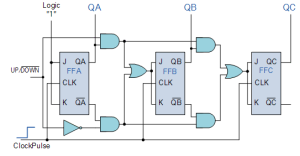Fig.5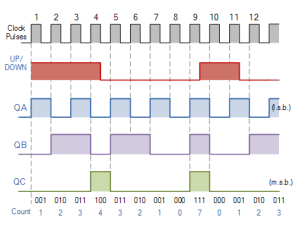The circuit above is of a simple 3-bit Up/Down synchronous counter using JK flip-flops configured to operate as toggle or T-type flip-flops giving a maximum count of zero (000) to seven (111) and back to zero again.

Hence, the 3-Bit counter advances upward in sequence (0,1,2,3,4,5,6,7) or downwards in reverse sequence (7,6,5,4,3,2,1,0).

#### Modulus Counter (MOD-N Counter)

The 2-bit ripple counter is called as MOD-4 counter and 3-bit ripple counter is called as MOD-8 counter. So in general, an n-bit ripple counter is called as modulo-N counter. Where,MOD number = 2n

#### TYPE OF MODULUS

• 2-bit up or down (MOD-4)
• 3-bit up or down (MOD-8)
• 4-bit up or down (MOD-16)

### Application of the counters

• Frequency counters
• Digital clock
• Time measurement
• A to D converter
• Frequency divider circuits
• Digital triangular wave generator